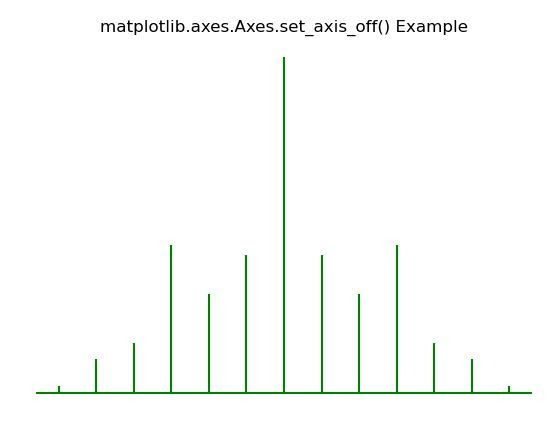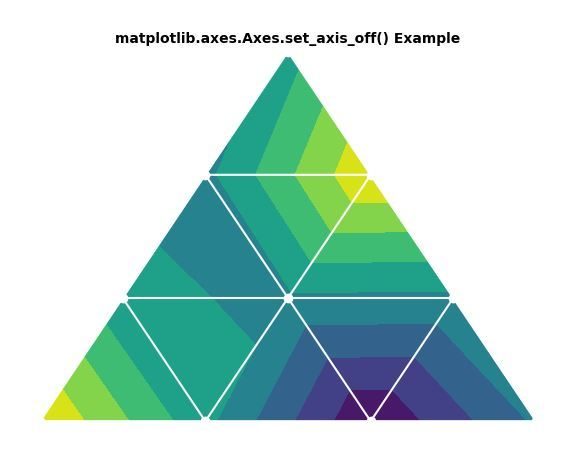# Matplotlib.axes.Axes.set_axis_off() in Python

Matplotlib is a library in Python and it is numerical – mathematical extension for NumPy library. The Axes Class contains most of the figure elements: Axis, Tick, Line2D, Text, Polygon, etc., and sets the coordinate system. And the instances of Axes supports callbacks through a callbacks attribute.

## matplotlib.axes.Axes.set_axis_off() Function

The Axes.set_axis_off() function in axes module of matplotlib library is used to turn the x- and y-axis off and this affects the axis lines, ticks, ticklabels, grid and axis labels.

Syntax: Axes.set_axis_off(self)

Parameters: This method does not accept any parameters.

Returns:This method does not returns anything.

Below examples illustrate the matplotlib.axes.Axes.set_axis_off() function in matplotlib.axes:

Example 1:

 `# Implementation of matplotlib function ` `import` `matplotlib.pyplot as plt ` `import` `numpy as np ` `   `  `# Time series data ` `geeksx ``=` `np.array([``24.40``, ``110.25``, ``20.05``,  ` `                  ``22.00``, ``61.90``, ``7.80``, ` `                   ``15.00``]) ` ` `  `geeksy ``=` `np.array([``24.40``, ``110.25``, ``20.05``, ` `                   ``22.00``, ``61.90``, ``7.80``, ` `                   ``15.00``]) ` `   `  `fig, ax ``=` `plt.subplots() ` `ax.xcorr(geeksx,  geeksy, maxlags ``=` `6``,  ` `         ``color ``=``"green"``) ` ` `  `ax.set_axis_off() ` ` `  `ax.set_title('matplotlib.axes.Axes.set_axis_off()\ ` `Example') ` `plt.show() `

Output:Example 2:

 `# Implementation of matplotlib function ` `import` `matplotlib.pyplot as plt ` `import` `matplotlib.tri as mtri ` `import` `numpy as np ` `    `  `x ``=` `np.asarray([``0``, ``1``, ``2``, ``3``, ``0.5``, ` `                ``1.5``, ``2.5``, ``1``, ``2``, ` `                ``1.5``]) ` ` `  `y ``=` `np.asarray([``0``, ``0``, ``0``, ``0``, ``1.0``,  ` `                ``1.0``, ``1.0``, ``2``, ``2``,  ` `                ``3.0``]) ` ` `  `triangles ``=` `[[``0``, ``1``, ``4``], [``1``, ``5``, ``4``],  ` `             ``[``2``, ``6``, ``5``], [``4``, ``5``, ``7``], ` `             ``[``5``, ``6``, ``8``], [``5``, ``8``, ``7``],  ` `             ``[``7``, ``8``, ``9``], [``1``, ``2``, ``5``],  ` `             ``[``2``, ``3``, ``6``]] ` ` `  `triang ``=` `mtri.Triangulation(x, y, triangles) ` `z ``=` `np.cos(``1.5` `*` `x) ``*` `np.cos(``1.5` `*` `y) ` `    `  `fig, axs ``=` `plt.subplots() ` `axs.tricontourf(triang, z) ` `axs.triplot(triang, ``'go-'``, color ``=``'white'``) ` ` `  `axs.set_axis_off() ` `axs.set_title('matplotlib.axes.Axes.set_axis_off()\ ` `Example``', fontsize = 10, fontweight ='``bold') ` `plt.show() `

Output:Whether you're preparing for your first job interview or aiming to upskill in this ever-evolving tech landscape, GeeksforGeeks Courses are your key to success. We provide top-quality content at affordable prices, all geared towards accelerating your growth in a time-bound manner. Join the millions we've already empowered, and we're here to do the same for you. Don't miss out - check it out now!

Previous
Next